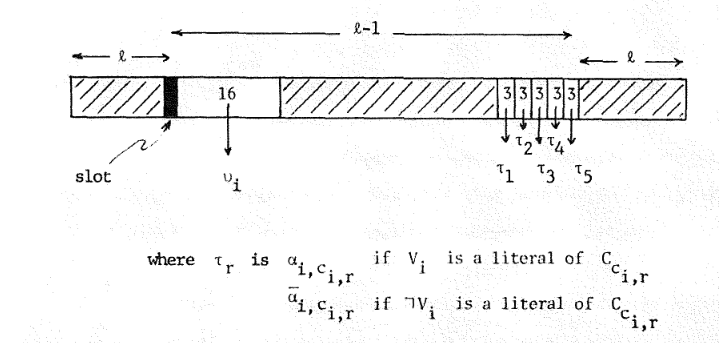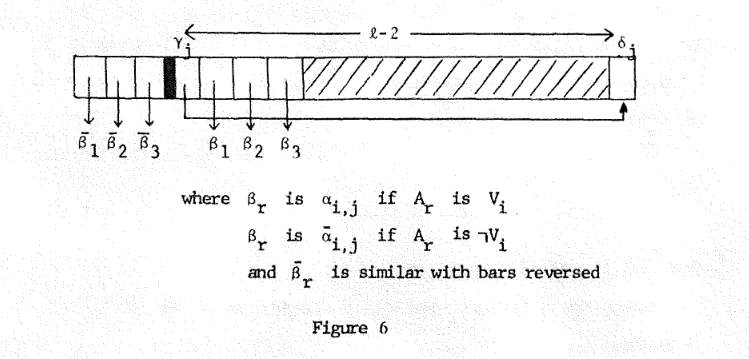# Tag Archives: Code Generation With Unfixed Variable Locations

## Code Generation With Unfixed Variable Locations

Sorry for the long break.  I’m hoping to use this summer to get most of the rest of the way through this book.

The Problem: Code Generation With Unfixed Variable Locations.  This is problem PO8 in the appendix.

The Description: Given a program (a sequence of instructions I1..In), a set V of variables, a “short address span” B, and a desired program size M.  We want to assign addresses to both the instructions of the program and the variables.  If an instruction accesses a variable that is within B instructions of its use, we can represent that instruction in one byte (using the “short address”).  Otherwise, the instruction is represented in two bytes (using the “long address”).  Can we place all instructions and variables in M addresses or less?

Example: Suppose I have the following instructions (A, B, and C are variables, “ref” just means the instruction refers to that variable)

```ref A
ref A
ref B
ref C
```

If B=1 (so all short references have to be within one instruction of the variable address), we can place all instructions and variables in 7 addresses, for example:

```ref A
A
ref A
ref B
B
C
ref C
```

If instead that second A reference was at the end of the sequence of instructions:

```ref A
ref B
ref C
ref A
```

..then (because we can’t change the order of the instructions in the program), we would have to make one of the references to A long, for example:

```ref A
A
ref B
B
C
ref C
ref A (long)
```

That second instruction that references A is a long reference, so this program needs 8 bytes of address space.

Reduction: The paper by Robertson uses 3SAT, So let’s start with a 3SAT instance with n variables and m clauses, each clause Cj contains literals Aj1, Aj2, and Aj3.Having just 1 or 2 literals in a clause is ok too.

Robertson notes that for any two literals V and W, if you have V and ~W appear as one (complete, two-element) clause, and also have ~V and W appear as a different clause, then V and W must both have the same truth value in order to satisfy both clauses.  This gets us a way to add more variables to the formula that have truth values the same as some other variable.  Specifically, if some variable V shows up in our formula more than 5 times, we can create a new variable W to replace three of those occurrences of V, and add two clauses to the end (the clauses (V ∨ ~W) and (~V ∨ W)).  This forces our new W to have the same truth value V will have if the formula is satisfied, and reduces the number of occurrences of V.  We repeat this process until no variable appears more than 5 times in the formula.  So, now we can say that for some variable Vi, it appears in clauses Ci1 through Ci5 (or maybe less than 5 if the variable occurs less than 5 times).

The program we will build will consist of “modules” that can be moved independently.  Each variable will have two modules (“t” and “f” modules), and each clause will have one.  Here is a picture of a variable “t” module:“l” is the paper’s version of B- the maximum distance we can put a short reference to a variable.

What we’re seeing here is:

• A “slot” we can put a variable (or more than one) into
• 16 instructions that refer to a new variable ui
• 3 instructions that reference each of the variables corresponding to the (at most 5) literals and clauses the variable can appear in.

The “f” mode is similar except that the definition of τr is negated (it’s the positive variable if ~Vi occurs as a literal).  The new variable ui appears in the t and f modules for variable i and noplace else, so the only way references to it can be short is if it is placed into the “slot” in either the “t” module (meaning variable i is set to true) or the “f” module (meaning variable i is set to false).  If we put ui in the slot of (say) the “f” module, then we have enough room to also place the variables corresponding to the literals in the same block making everything short.  But by putting the ui variable in the “f” module, all of the references to it from the “t” module will be long (adding 16 extra bytes of address space), making all of the literals too far away from the slot for the slot to be useful in that module.

We also have a module for the clause (the “c”-module):Notice that the versions of the literals that actually appear in the clause are to the right of the slot.  These α variables are the same ones we had in the variable modules (so each α variable shows up once in a “c” module, but 3 times each in a “t” or “f” module).  Since there are more references to the α variables in our variable modules, the optimal placement should be to place those variables in the slot of the “t” or “f” modules (not in the “c” module).  The “remaining” α variables (the negations of the ones we put into the slot in either the “t” or “f” modules and which it didn’t help us to put in the slot of the other module) can go into the slot here and make one of the corresponding β references short.

We do have that extra γj cell to play around with, and here is where either the paper has a mistake, or I lose the ability to follow.  What is supposed to happen is that if we fill the slot with a variable that is referred to by one of the β variables then that reference will be short, and the reference from γ to δ will be within the range of a short reference.  That’s fine, but the paper wants this to be the α variable that we did not place in the “t” or “f” module.  But if placing the α in the “t” module meant setting the variable to true, then what is not in the module is the negation of that module, which feels like we are doing things backward- like we want to set the variable to true, we should have put ui in the “f” module so that the literal that can be placed in our clause module corresponds to the true version of the literal.

I’m not sure it matters much to the reduction.  If I’m right, it just means we should have labeled the α variables the negations of what they really are.  But it does worry me.

The proof finishes with deciding values of B and M to make it all work.  B ends up being 31 (it comes from the distance we need to separate the 16 ui instructions from the τ instructions), and M ends up being 16n+4* the total number of literals in the formula.

Difficulty: 7.  This has a lot of pieces, but (except for that end part) I think it’s pretty feasible to see how all of the pieces fit together.